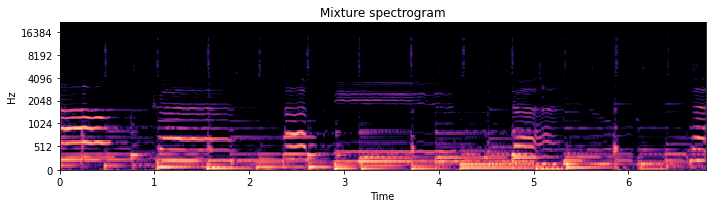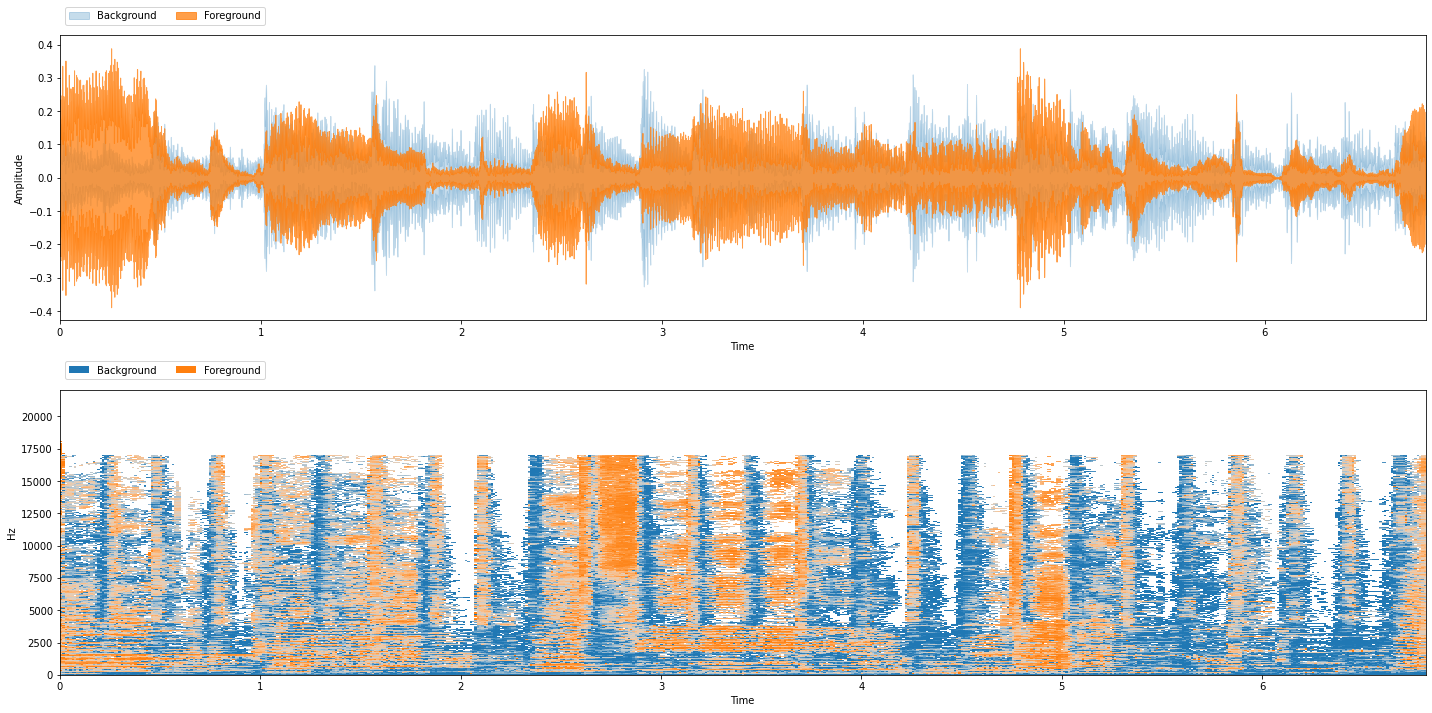# Putting it All Together: Repetition¶

So far, we have learned about the concepts behind source separation, but now let’s put everything together and get a feel for how the ideas all work in practice. In this section, we will synthesize all the ideas of the previous sections; we will examine and compare three similar algorithms on the same song. We hope to provide you with some intuition about how all the pieces fit together.

## Repetition¶

Repetition is a common feature of almost every type of music. Oftentimes, we can use this cue to identify different sources in a song: a trumpet might improvise a new melody over a backing band that is repeating the same few bars. In this case, we can leverage the repetition of the backing band to isolate the trumpet.

With that in mind, we will explore three algorithms that attempt to separate a repeating background from a non-repeating foreground. The basic assumptions here are:

1. that there is repetition in the mixture, and

2. the repetition captures what we want to separate.

These assumptions hold quite well if we want to separate a trumpet from a backing band, but might not work if we want to isolate a drum set from the rest of the band because the drum set is usually playing a repeating pattern.

### Setup¶

The three algorithms we will look at in this section all input magnitude spectrograms from a mixture, try to find the repeating parts in the mixture, and separate them out by creating a mask for the foreground and background. Here we’ll attempt to try to separate a singer from the background instruments.

### REPET Overview¶

The first algorithm we will explore here is called the REpeating Pattern Extraction Technique or REPET [RP12b]. REPET works like this:

1. Find a repeating period, $$t_r$$ seconds (e.g., the number of seconds which a chord progression might start over).

2. Segment the spectrogram into $$N$$ segments, each with $$t_r$$ seconds in length.

3. “Overlay” those $$N$$ segments.

4. Take the median of those $$N$$ stacked segments and make a mask of the median values.

We’ll use REPET to demonstrate how to run a source separation algorithm in nussl.

%%capture
!pip install git+https://github.com/source-separation/tutorial

# Do our imports
import warnings
warnings.simplefilter('ignore')
import nussl
import matplotlib.pyplot as plt
import numpy as np
from pprint import pprint
from common import viz


### Our Input Mixture¶

Let’s download an audio file that has a lot of repetition in it, and inspect and listen to it:

# This example is from MUSDB18. We will discuss this in a later section.
item = musdb

# Get the mix and sources
mix = item['mix']
sources = item['sources']

# Listen to the audio
mix.embed_audio()

# Visualize the spectrogram
plt.figure(figsize=(10, 3))
plt.title('Mixture spectrogram')
nussl.utils.visualize_spectrogram(mix, y_axis='mel')
plt.tight_layout()
plt.show()### Using REPET in nussl¶

Now we need to instantiate a Repet object in nussl. We can do that like so:

repet = nussl.separation.primitive.Repet(mix)


Now the repet object has our AudioSignal, it’s easy to run the algorithm:

# Background and Foreground Masks

<class 'nussl.core.masks.soft_mask.SoftMask'>


Oh, look! The repet object returned masks! Woohoo!

Now that we have masks, we can apply them to the mix to get source estimates.

#### Without Phase¶

Let’s apply the masks to the mixture spectrogram without considering the phase.

# Get the mask numpy arrays

# Multiply the masks to the magnitude spectrogram
mix.stft()
mix_mag_spec = mix.magnitude_spectrogram_data

# Make new AudioSignals for background and foreground without phase
bg_no_phase = mix.make_copy_with_stft_data(bg_no_phase_spec)
_ = bg_no_phase.istft()
fg_no_phase = mix.make_copy_with_stft_data(fg_no_phase_spec)
_ = fg_no_phase.istft()


Let’s hear what these source estimates sound like:

print('REPET Background without Phase')
_ = bg_no_phase.embed_audio()

print('REPET Foreground without Phase')
_ = fg_no_phase.embed_audio()

REPET Background without Phase

REPET Foreground without Phase


Not bad, but… not great! The phase artifacts are much more apparent in the foreground.

#### With the Mixture Phase¶

Let’s apply the mixture phase to our estimates. We can use the function we talked about earlier:

def apply_mask_with_noisy_phase(mix_stft, mask):
mix_magnitude, mix_phase = np.abs(mix_stft), np.angle(mix_stft)
src_stft = src_magnitude * np.exp(1j * mix_phase)
return src_stft

# Make new AudioSignals for background and foreground with phase
bg_phase = mix.make_copy_with_stft_data(bg_stft)
_ = bg_phase.istft()
fg_phase = mix.make_copy_with_stft_data(fg_stft)
_ = fg_phase.istft()


Again, let’s hear the results:

print('REPET Background with Phase')
_ = bg_phase.embed_audio()

print('REPET Foreground with Phase')
_ = fg_phase.embed_audio()

REPET Background with Phase

REPET Foreground with Phase


Much better!

### The Easy Way¶

nussl provides functionality for all of the issues regarding applying the mask by doing one of the following:

# Will make AudioSignal objects after we're run the algorithm
repet = nussl.separation.primitive.Repet(mix)
repet.run()
repet_bg, repet_fg = repet.make_audio_signals()

# Will run the algorithm and return AudioSignals in one step
repet = nussl.separation.primitive.Repet(mix)
repet_bg, repet_fg = repet()


### Evaluation¶

Okay, now let’s evaluate how well our REPET model did. First we will inspect the model’s output:

viz.show_sources({'Background': repet_bg, 'Foreground': repet_fg})